# The GCAL model definition

The GCAL model (gain control, adaptation and lateral) is a general purpose cortical developmental model of topographic map formation in the primary visual cortex (V1), driven by the presentation of visual patterns on a retinal sheet. This model accounts for receptive field formation, the emergence and of orientation selectivity across the cortical surface as well as contrast-invariant orientation tuning. The GCAL model is robust to large changes in the contrast of the training patterns that may flucuate between widely varying statistical distributions. The resulting orientation maps are robust and stable yet adaptive development that reflects the statistics of the input.

The GCAL model and related submodels (L,AL and GCL) are fully described by Stevens et al. (2013):

@article{Stevens2013,
author = {Stevens, Jean-Luc R. and Law, Judith S. and
Antol\'{i}k, J\'{a}n and Bednar, James A.},
title = {Mechanisms for Stable, Robust, and Adaptive
Development of Orientation Maps in the Primary Visual Cortex},
journal = {The Journal of Neuroscience},
volume = {33},
number = {40},
pages = {15747-15766},
year = {2013},
doi = {10.1523/JNEUROSCI.1037-13.2013},
url = {http://www.jneurosci.org/content/33/40/15747.full}
}


If you can double-click on this text and edit it, you are in a live IPython Notebook environment where you can run the code and explore the model. Otherwise, you are viewing a static (e.g. HTML) copy of the notebook, which allows you to see the precomputed results only. To switch to the live notebook, see the notebook installation instructions.

This IPython notebook constructs the definition of the GCAL model and related submodels (L,AL and GCL), for use with the Topographica simulator. The resulting model file is used by the accompanying notebook to launch all the necessary simulations and collate the necessary data to plot all the figures published in Stevens et al. (2013).

Running this notebook defines the model file source (gcal.ty) while the model is loaded into memory for inspection, allowing you to see how the model components are specified and put together. Examples of the retinal training patterns and model weigths are shownn as well as the orientation map after running 3000 iterations.

A static version of this notebook may be viewed online. To run the live notebook and explore the model interactively, you will need both IPython notebook and Topographica. The installation instructions and software dependencies are listed here

Note that the gcal.ty model file exported by the notebook may be run without IPython using Topographica as follows:

./topographica -g ./gcal.ty

Once you have exported the gcal.ty file, you can also conveniently load the model into Topographica and launch the GUI from here. Note that you will need topo on the path - the following code cell imports the modules needed in this notebook and adds topo to the path, assuming you are running this notebook in the stevens.jn13 directory:

In :
import os, sys, math
sys.path += [os.path.abspath(os.path.join('..','..'))]

import topo
import imagen
import lancet
import pandas

from jn13_figures.lib import misc


## Contents

Explore the model
Load the model and explore it interactively. It takes approx. 7 minutes to run the full 20000 iterations on an intel i5.
Model Utilities
Utilities for display and for approximating the goggle rearing condition.
The docstring for the model file and the list of Python imports.
Model Parameters
The parameters used to specify the L, AL, GCL, and GCAL models.
Training patterns
The gaussian, noisy disk, image, and blurred retinal images used for training.
Sheet definitions
Defining the neural sheets in the model - photoreceptor, LGN layers, and V1.
Projections
Defining the synaptic connections between neurons in each sheet and between sheets.
Export
Setting up the layout in the GUI, default measurement settings, and exporting the definition to gcal.ty
Inspection
A look at the model after 3000 iterations, including measurement of an orientation map.

## Explore the model with the GUI

1. To step through development, open the activity window (Plots -> Activity) and click 'Go'
2. To measure an OR map, open Plots -> Preference Maps -> Orientation Preference then click the top blue arrow.
In :
prompt_msg = 'Would you like to explore the GCAL model with the Topographica GUI? (y/n): '

if (raw_input(prompt_msg) if True else 'n') == 'y': # Set False to disable prompt.
misc.open_gui()
if os.path.isfile('gcal.ty'):
execfile('gcal.ty')
else:

Would you like to explore the GCAL model with the Topographica GUI? (y/n): n



# Model definition

This section includes some utilities used later in this notebook, the model docstring and imports and the model parameters. Anything marked __GCAL_TY__ will go to the .ty file later,

In :
__GCAL_TY__ = True # Code cells marked at the top with this identifier will be exported to gcal.ty


### Description and imports

In :
__GCAL_TY__
# -*- mode: python;-*-
"""
GCAL

Simple but robust single-population V1 model orientation map from:

Jean-Luc R. Stevens, Judith S. Law, Jan Antolik, and James A. Bednar.
Mechanisms for stable, robust, and adaptive development of orientation
maps in the primary visual cortex.

@article{Stevens02102013,
author = {Stevens, Jean-Luc R. and Law, Judith S. and Antolik, Jan and Bednar, James A.},
title = {Mechanisms for Stable, Robust, and Adaptive Development of Orientation Maps in the Primary Visual Cortex},
journal = {The Journal of Neuroscience}
volume = {33},
number = {40},
pages = {15747-15766},
year = {2013},
doi = {10.1523/JNEUROSCI.1037-13.2013},
url = {http://www.jneurosci.org/content/33/40/15747.full}
}
"""

from math import pi
import os

import numpy
import param

from topo import learningfn,numbergen,transferfn,pattern,projection,responsefn,sheet

import topo.learningfn.optimized
import topo.learningfn.projfn
import topo.transferfn.optimized
import topo.pattern.random
import topo.pattern.image
import topo.responsefn.optimized
import topo.sheet.lissom
import topo.sheet.optimized

import topo.transferfn.misc
from topo.base.arrayutil import DivideWithConstant
from topo.transferfn.misc import HomeostaticResponse
from topo.misc.commandline import global_params as p


### Model parameters

These are the parameters that define the quality of the simulation, the exact model loaded (L,AL,GCL or GCAL), the dataset used and other key model and training parameters:

In :
__GCAL_TY__

input_seed = param.Integer(default=42, doc="""
The random seed to set the input patterns"""),

dataset=param.ObjectSelector(default='Gaussian',objects=
['Gaussian','Nature', 'VGR'],doc="""
Set of input patterns to use::
:'Gaussian': Two-dimensional Gaussians
:'Nature':   Shouval's 1999 monochrome 256x256 images.
:'VGR':       Simulated vertical goggle rearing
(anisotropically blurred Shouval)"""),

num_inputs=param.Integer(default=2,bounds=(1,None),doc="""
How many input patterns to present per unit area at each
iteration, when using discrete patterns (e.g. Gaussians)."""),

aff_strength=param.Number(default=1.5,bounds=(0.0,None),doc="""
Overall strength of the afferent projection to V1."""),

exc_strength=param.Number(default=1.7,bounds=(0.0,None),doc="""
Overall strength of the lateral excitatory projection to V1."""),

inh_strength=param.Number(default=1.4,bounds=(0.0,None),doc="""
Overall strength of the lateral inhibitory projection to V1."""),

aff_lr=param.Number(default=0.1,bounds=(0.0,None),doc="""
Learning rate for the afferent projection to V1."""),

exc_lr=param.Number(default=0.0,bounds=(0.0,None),doc="""
Learning rate for the lateral excitatory projection to V1."""),

inh_lr=param.Number(default=0.3,bounds=(0.0,None),doc="""
Learning rate for the lateral inhibitory projection to V1."""),

area=param.Number(default=1.0,bounds=(0,None),
inclusive_bounds=(False,True),doc="""
Linear size of cortical area to simulate.
2.0 gives a 2.0x2.0 Sheet area in V1."""),

retina_density=param.Number(default=24.0,bounds=(0,None),
inclusive_bounds=(False,True),doc="""
The nominal_density to use for the retina."""),

lgn_density=param.Number(default=24.0,bounds=(0,None),
inclusive_bounds=(False,True),doc="""
The nominal_density to use for the LGN."""),

cortex_density=param.Number(default=49.0,bounds=(0,None),
inclusive_bounds=(False,True),doc="""
The nominal_density to use for V1."""),

# Noisy disk parameters

retinal_waves=param.Integer(default=0,bounds=(0,None),doc="""
How many retinal wave (noisy disk) presentations before the
chosen dataset is displayed. Zero for no noisy disk
presentations otherwise 6000 has been typically used.."""),

percent_noise = param.Number(default=50, doc="""
The percentage of the noise strength to the disk strength for noisy disks"""),

contrast=param.Number(default=20, bounds=(0,100),
inclusive_bounds=(True,True),doc="""
Brightness of the input patterns as a contrast (percent)."""),

# Toggle between the L, GCL, AL and GCAL models

gain_control = param.Boolean(default=True, doc="""
Whether or not gain-control (divisive lateral inhibitory) is to be
applied in the LGN"""),

homeostasis = param.Boolean(default=True, doc="""
Whether or not the homeostatic adaption should be applied in V1"""),

t_init = param.Number(default=0.20, doc="""
The initial V1 threshold value. This value is static in the L and GCL models
and adaptive in the AL and GCAL models.""")
)


## Training patterns

On every training iteration, a stimulus is presented on the retina. To demonstrate the robustness of the GCAL model, we used four types of image:

Gaussian patches
Elongated Gaussians displayed with uniform random orientation and position
Image Patches
Natural image patches to present to the GCAL model (figure 10 and 12)
Goggle Rearing
Approximation to vertical goggle rearing achieved by anisotropically blurring the image patch dataset.
Noisy Disks
Noisy disk stimuli roughly approximating retinal waves for 6000 iterations (figures 10, 11, 12)
In :
__GCAL_TY__
### Input patterns

# Scale of 1.0 is equivalent to 100% contrast.
contrast_scale = p.contrast / 100.0
if p.dataset=="Gaussian":
total_num_inputs=int(p.num_inputs*p.area**2)
inputs=[pattern.Gaussian(x=numbergen.UniformRandom(lbound=-(p.area/2.0+0.25),
ubound= (p.area/2.0+0.25),seed=p.input_seed+12+i),
y=numbergen.UniformRandom(lbound=-(p.area/2.0+0.25),
ubound= (p.area/2.0+0.25),seed=p.input_seed+35+i),
orientation=numbergen.UniformRandom(lbound=-pi,ubound=pi,seed=p.input_seed+21+i),
size=0.088388, aspect_ratio=4.66667, scale=contrast_scale)
for i in xrange(total_num_inputs)]
combined_inputs = pattern.SeparatedComposite(min_separation=0,generators=inputs)


### * First three default training stimuli (Gaussians)*

In :
misc.view_patterns(combined_inputs,radius=p.area/2.0+0.25+0.375+0.5)

0 1 2
patterns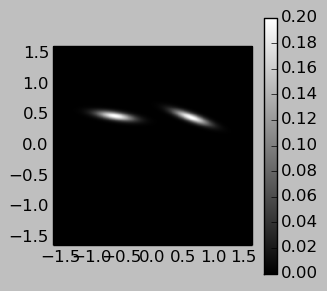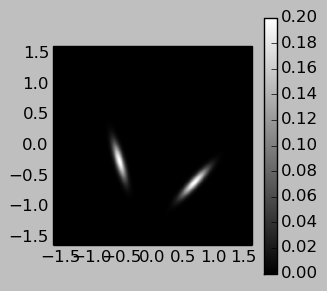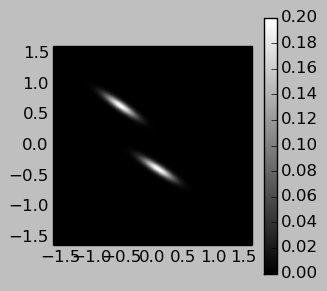### * First three unrotated and unscaled natural image patches (Shouval et al)*

In :
images_dir = param.resolve_path('images', path_to_file=False)
images = lancet.FilePattern('Nature', os.path.join(images_dir, 'shouval','combined*.png'))

0 1 2
Nature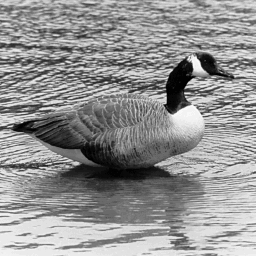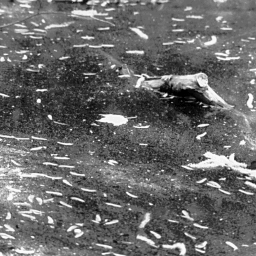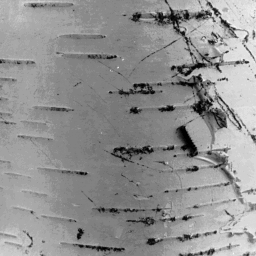### Convolution test using an isotropic kernel (shown on the left):

In :
isotropic_kernel = imagen.Gaussian(aspect_ratio=1.0, xdensity=128, ydensity=128,
size=0.05, orientation=math.pi/2.0)
misc.generate_GR(images_dir, isotropic_kernel, name='blur')
images = lancet.FilePattern('blur', os.path.join(images_dir, 'blur','combined*_blur.png'))
lancet.ViewFrame(convolve_dframe.T)

0 0 1
blur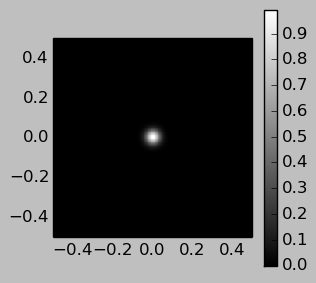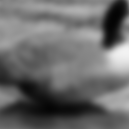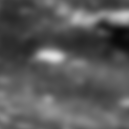### Example of anistropically blurred image used (kernel shown on the left):

In :
misc.generate_GR(images_dir, name='VGR')
images = lancet.FilePattern('VGR', os.path.join(images_dir, 'VGR','combined*_VGR.png'))
lancet.ViewFrame(anisotropic_dframe.T)

0 0 1
VGR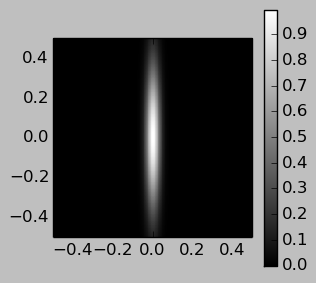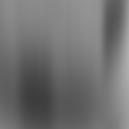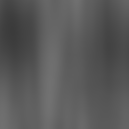### Model file definition for image patches and google rearing condition:

In :
__GCAL_TY__
if p.dataset in ["Nature", "VGR"]:
# Do not randomly rotate GR patches (otherwise horizontal bias is meaningless)
patch_orientation = 0.0 if p.dataset=="VGR" else numbergen.UniformRandom(lbound=-pi,ubound=pi,seed=p.input_seed+65)

if p.dataset=="Nature":
image_filenames= [param.resolve_path("images/shouval/combined%02d.png"%(i+1)) for i in xrange(25)]
else:
# Actual ordering used in the paper for historical reasons,
# makes little difference if ordering=range(1,26) is used instead.
ordering = [1,25,8,24,22,23,4,15,17,13,5,20,18,14,3,6,12,10,21,7,9,2,16,11,19]
image_filenames  = [param.resolve_path("images/VGR/combined%02d_VGR.png" % i) for i in ordering]

inputs=[pattern.image.FileImage(filename=f,
scale=contrast_scale,
size=10.0,
x=numbergen.UniformRandom(lbound=-0.75,ubound=0.75,seed=p.input_seed+12),
y=numbergen.UniformRandom(lbound=-0.75,ubound=0.75,seed=p.input_seed+36),
orientation=patch_orientation)  for f in image_filenames]

combined_inputs = pattern.Selector(generators=inputs)


### Model file definition for noisy disk patterns:

In :
__GCAL_TY__

noise_ratio = (p.percent_noise / 100.0)
disk_scale= 1.0 / (1.0 + noise_ratio)
rand_scale= noise_ratio / (1.0 + noise_ratio)

scale=contrast_scale,
generators=[topo.pattern.Disk(
x=numbergen.UniformRandom(lbound=-2.125,ubound=2.125, seed=p.input_seed+12),
y=numbergen.UniformRandom(lbound=-2.125,ubound=2.125, seed=p.input_seed+36),
size=2.0, aspect_ratio=1.0, scale = disk_scale,

topo.pattern.random.UniformRandom(scale=rand_scale)])]

retina_inputs = topo.pattern.Selector(generators=disks_inputs)

if p.retinal_waves == 0:
retina_inputs = combined_inputs
else:
topo.sim.schedule_command(p.retinal_waves, 'topo.sim["Retina"].set_input_generator(combined_inputs, push_existing=False)')


### First three examples of noisy disk patterns used to approximate reitnal wave stimuli:

In :
misc.view_patterns(topo.pattern.Selector(generators=disks_inputs), radius=p.area/2.0+0.25+0.375+0.5)

0 1 2
patterns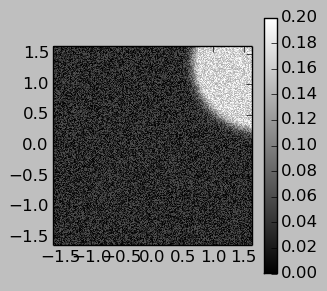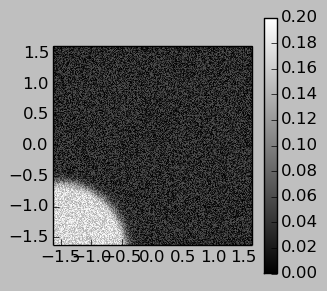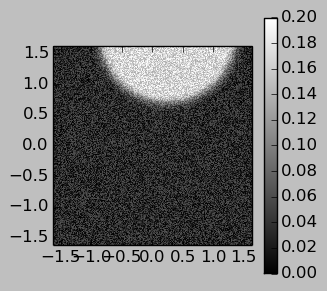#### Enabling optimizations and making CFs circular

In :
__GCAL_TY__
### Specify weight initialization, response function, and learning function
projection.CFProjection.cf_shape=pattern.Disk(smoothing=0.0)
projection.CFProjection.response_fn=responsefn.optimized.CFPRF_DotProduct_opt()
projection.CFProjection.learning_fn=learningfn.optimized.CFPLF_Hebbian_opt()
projection.CFProjection.weights_output_fns=[transferfn.optimized.CFPOF_DivisiveNormalizeL1_opt()]
projection.SharedWeightCFProjection.response_fn=responsefn.optimized.CFPRF_DotProduct_opt()


# Sheet Definitions

Retina Sheet
The photoreceptor sheet that represents the retina onto which the visual image is projected.
LGN Sheets
The two sheets that represent the lateral geniculate nucleus (center-surround receptive fields)
V1 Sheet
Sheet representing the primary visual cortex